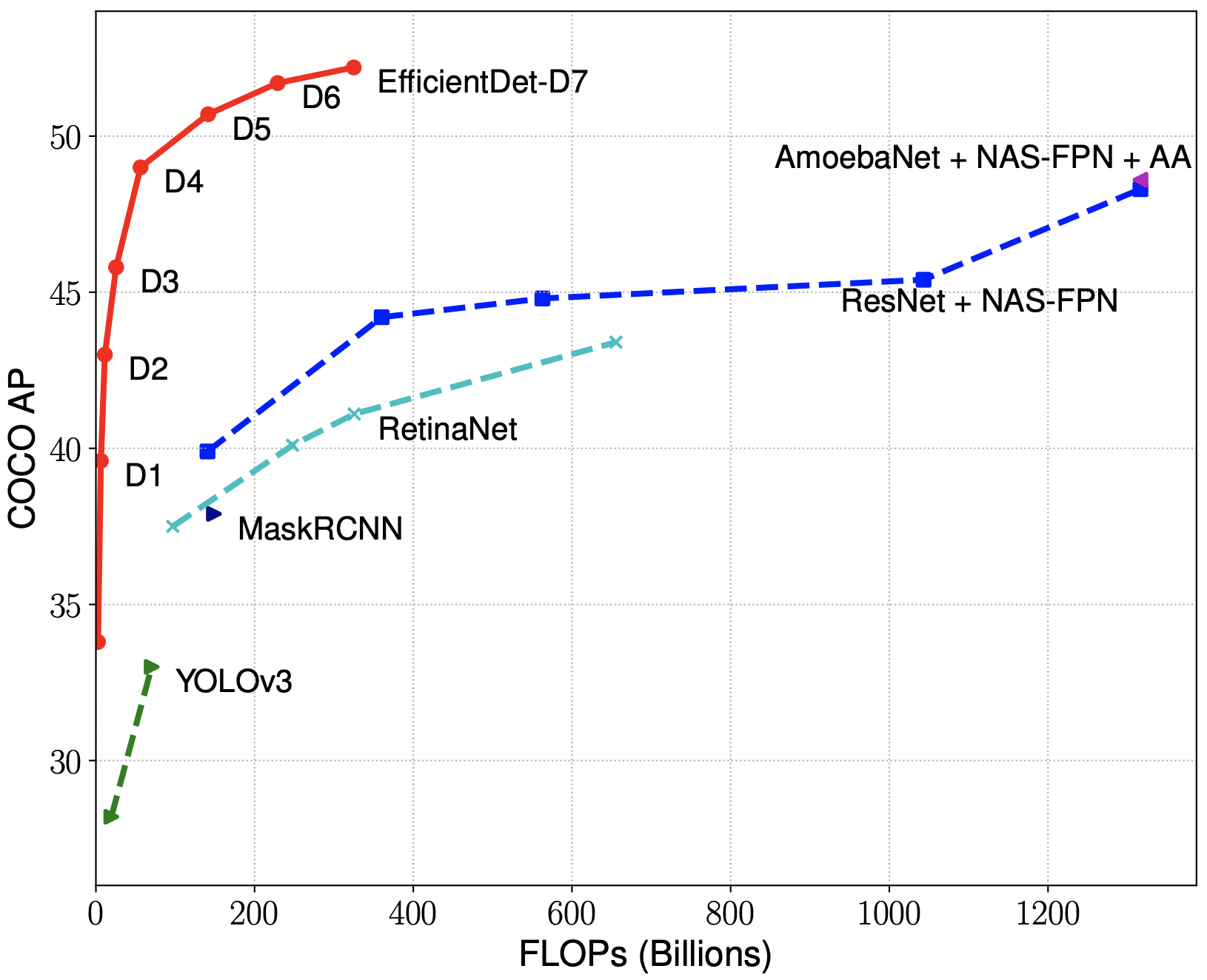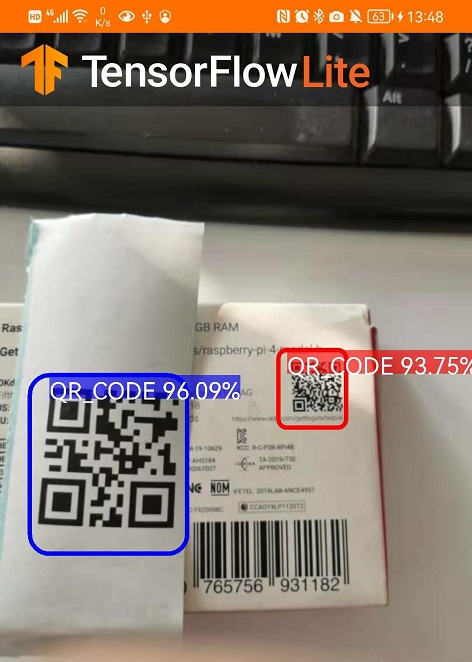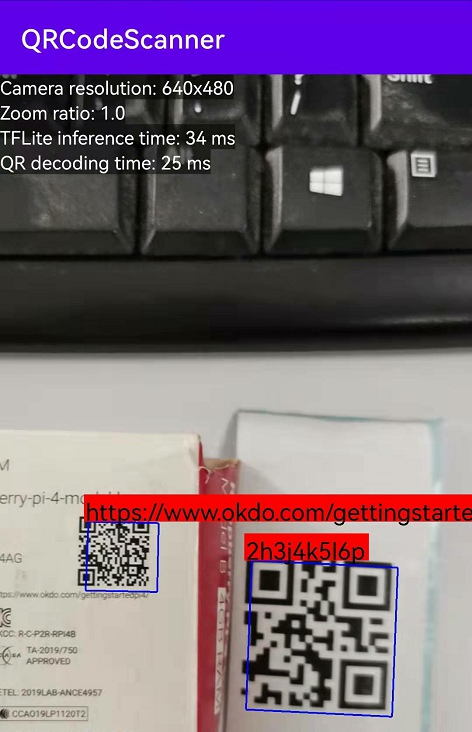×

# Android QR Code Detection with TensorFlow Lite

A week ago, I made an experiment to test the QR code detection performance with YOLOv4 on Android. The inference time of running the YOLOv4 model on CPU is more than 100ms, which does not reach my expectation. Since Android Neural Networks API (NNAPI) provides hardware acceleration for TensorFlow Lite models, I decided to train a TensorFlow Lite model to speed up the inference time.

## Steps to Train QR Code Detection Model with TensorFlow Lite Model Maker

1. Install TensorFlow Lite Model Maker:

`````` pip install tflite-model-maker
``````
2. Get the GitHub repository that contains the image set:

`````` git clone https://github.com/yushulx/barcode-qrcode-images.git
``````
3. Partition the image set into training and validation sets:

`````` cd tensorflow-lite
python partition_dataset.py -x -i ../images -r 0.1 -o ./
``````
4. According to the TensorFlow tutorial, create the following Python script to train the model:

`````` from tflite_model_maker.config import QuantizationConfig
from tflite_model_maker.config import ExportFormat
from tflite_model_maker import model_spec
from tflite_model_maker import object_detector

import tensorflow as tf
assert tf.__version__.startswith('2')

tf.get_logger().setLevel('ERROR')
from absl import logging
logging.set_verbosity(logging.ERROR)

spec = model_spec.get('efficientdet_lite0')
train_data = object_detector.DataLoader.from_pascal_voc(images_dir="train", annotations_dir="train", label_map={1: "QR_CODE"} )
validation_data = object_detector.DataLoader.from_pascal_voc(images_dir="test", annotations_dir="test", label_map={1: "QR_CODE"} )
model = object_detector.create(train_data, model_spec=spec, batch_size=8, train_whole_model=True, validation_data=validation_data)
model.export(export_dir='.')
``````

EfficientDet-D0 has comparable accuracy as YOLOv3.Once the model is saved, we can download the TensorFlow Lite example and replace the model file with the one we just created.

To make the model work:

1. Drag the `model.tflite` file to assets folder.
2. Change `TF_OD_API_MODEL_FILE` to `model.tflite` in `DetectorActivity.java`:

`````` private static final String TF_OD_API_MODEL_FILE = "model.tflite";
``````
3. Clear `labelmap.txt` and add the following line:

`````` QR_CODE
``````

So far, the QR code detection model is ready to be used. We can test it on the Android device.## QR Code Scanner with TensorFlow Lite

Now, let’s integrate TensorFlow Lite API into our Android QR Code Scanner.

1. Copy `model.tflite` and `labelmap.txt` to assets folder.
2. Add the TensorFlow Lite library to `build.gradle`:

`````` // Import the Task Vision Library dependency (NNAPI is included)
``````
3. Copy `Detector.java` and `TFLiteObjectDetectionAPIModel.java` from the TensorFlow Lite example project to our project. Use NNAPI delegate for TensorFlow Lite:

`````` private TFLiteObjectDetectionAPIModel(Context context, String modelFilename) throws IOException {
optionsBuilder = ObjectDetectorOptions.builder();
optionsBuilder.setBaseOptions(BaseOptions.builder().useNnapi().build());
objectDetector = ObjectDetector.createFromBufferAndOptions(modelBuffer, optionsBuilder.build());
}
``````
4. Initialize the model detector in `CameraXActivity.java`:

`````` // Configuration values for the prepackaged QR Code model.
private static final int TF_OD_API_INPUT_SIZE = 416;
private static final boolean TF_OD_API_IS_QUANTIZED = true;
private static final String TF_OD_API_MODEL_FILE = "model.tflite";
private static final String TF_OD_API_LABELS_FILE = "labelmap.txt";
// Minimum detection confidence to track a detection.
private static final float MINIMUM_CONFIDENCE_TF_OD_API = 0.5f;
private Detector detector;
int cropSize = TF_OD_API_INPUT_SIZE;

private void initTFDetector() {
try {
detector =
TFLiteObjectDetectionAPIModel.create(
this,
TF_OD_API_MODEL_FILE,
TF_OD_API_LABELS_FILE,
TF_OD_API_INPUT_SIZE,
TF_OD_API_IS_QUANTIZED);
cropSize = TF_OD_API_INPUT_SIZE;
} catch (final IOException e) {
e.printStackTrace();
Toast toast =
Toast.makeText(
getApplicationContext(), "Detector could not be initialized", Toast.LENGTH_SHORT);
toast.show();
finish();
}
}
``````
5. In the camera frame callback function, convert `ImageProxy` to `Bitmap`:

`````` analysisUseCase.setAnalyzer(cameraExecutor,
imageProxy -> {
Bitmap bitmap = ImageUtils.getBitmap(imageProxy);
imageProxy.close();
});
``````

Note: the bitmap has been rotated, so we don’t need to rotate the coordinates of the QR code bounding box for rendering overlay.

To understand the implementation of `getBitmap()`, please refer to BitmapUtils.java.

6. Detect QR code and draw bounding boxes:

`````` final List<Detector.Recognition> tfResults = detector.recognizeImage(bitmap);
if (tfResults.size() > 0) {
for (final Detector.Recognition tfResult : tfResults) {
final RectF location = tfResult.getLocation();
if (location != null && tfResult.getConfidence() >= MINIMUM_CONFIDENCE_TF_OD_API) {
}
}
}
``````
7. Decode QR code with Dynamsoft Barcode Reader API:

`````` TextResult[] results = null;
try {
settings.barcodeFormatIds = EnumBarcodeFormat.BF_QR_CODE;
settings.expectedBarcodesCount = tfResults.size();
e.printStackTrace();
}
``````
8. Finally, test the QR code detection model on the Android device.We can see running TensorFlow Lite model to scan QR code is faster than running YOLOv4, but still slower than purely running Dynamsoft Barcode Reader.

## Source Code

https://github.com/yushulx/android-camera2-preview-qr-code-scanner